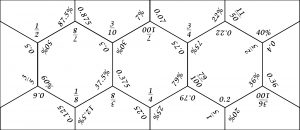# Worksheets on Number Topics

by | Jun 4, 2020 | 2nd level, 3rd level, Number Skills | 0 commentsAuthor: Emma Elley, Maths/physics teacher, Moray House School of Education and Sport

These worksheets will be suitable for pupils aged approximately from P6 to S2.

• Fractions/Decimal/Percentages hexagon dominoes: a JPEG to print out and cut out (see picture on right).
• Cut the pieces out, mix them up
• One way of indicating whether the learner is successful is to see if the shape that they recreate is a rectangle.
• Worksheet in Microsoft Word format: Negative numbers and subtraction of decimals
• Diagram in PowerPoint format showing Equivalent Fractions
• this could be used as a resource to use to help with working during an equivalent fraction lesson
• an alternative possible learning activity could be to ask the learner to recreate the diagram accurately on squared paper (using a width of 24 squares would be helpful).  Possible questions to go along with this could include:
• Why do all the eighths have to be the same size as each other?
• How are you working out how big to make the boxes in each row?
• How many 1/12s are equivalent to 1/6?
• What does the last row represent?
• What are 2 fractions that are equivalent to 1/8?